## Понедельник 23 июня, 14-00, ауд. 106

Понедельник, 23 июня, ауд. 106. Начало в 14:00.

Докладчик: Ville Salo (University of Turku).

Тема: Defining subshifts with multiheaded finite state automata on finitely generated groups.

### Abstract

If G is a finitely generated group, and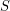a finite alphabet, we call a subset of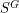a subshift if it is defined by a set of finite forbidden patterns (which may not be seen anywhere in the colored Cayley graph), and if the set of forbidden patterns is enumerated by a Turing machine, we say the subshift is computable. Given a finitely generated group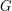, we can define a set of (computable) subshifts on it by multiheaded automata, by forbidding all finite patterns where the automaton halts (after the heads have walked around for some number of steps on the Cayley graph). For each finitely generated group(such as a finite group,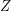,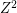,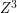or a free group), we ask the following questions: 1) Does there exist n such that every computable subshift ofis defined by a multiheaded finite state automaton with n heads? 2) If yes, what is the least such n for a given group? We characterize the groups on which the answer to question 1 is "yes", and for all such groups, show that the answer to question 2 is at most 3. This class includes many of the familiar examples, such as all groups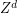and finitely generated free groups. We show that on, the answer to question 2 is precisely "3", but leave the question open for the groupsand. This is joint work with Ilkka Törmä.